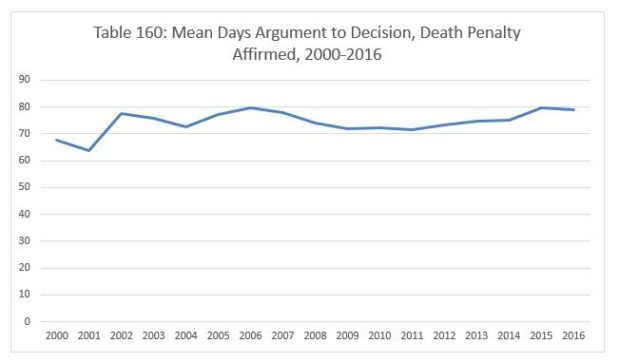For the past few weeks, we’ve been taking a close look at the California Supreme Court’s automatic death penalty appeals docket.  Yesterday, we calculated the average time from appointment of counsel to the scheduled oral argument and looked at whether the total time under submission told us anything about what the ultimate result would be.  Today, we turn to a related subject: how long does the average death penalty appeal remain pending after oral argument?

In Table 159 below, we report the mean time in days from oral argument to decision in death penalty appeals between 2000 and 2016.  With the exception of 2015, the yearly average has been quite stable, between 60 and 80 days each year.  In 2000, the average was 67.6 days.  In 2001, the average dropped to 62.91 days.  The average stayed in the 70s from 2002 through 2008 (77.43, 76.45, 73.1, 77.15, 79.53, 78.26 and 73.12 days).  In 2009, the Court averaged 69.8 days pending.  The average was between 70 and 75 days for the next five years.  In 2010, the number was 72.13 days.  In 2011, it was 72.38.  In 2012, it was 73.32.  In 2013, it was 74.61, and in 2014, the average was 73.91 days.  In 2014, we saw a one year jump to 126.35 days.  Finally, in 2016, the Court’s average was 79.67 days.The average time under submission for the entire period in cases where the Court reversed the death penalty was 100 days.  Cases in which the penalty was affirmed were typically about one third quicker.  In 2000 and 2001, the average was below 70 days – 67.6 and 63.7 days.  The Court’s average lag time remained in the 70s for each of the fourteen years that followed.  In 2002, the average was 77.38 days.  In 2003, it was 75.88.  In 2004, it was 72.47.  In 2005, it was 77.15.  In 2006, the average was 79.53 days.  In 2007, the average was 77.91.  In 2008, the average was 74.04.  In 2009, the average was 71.7 days.  In 2010, the average was 72.13 days.  In 2011, the average was 71.68 days.  In 2012, the average was 73.38 days.  In 2013, the average was 74.61 days.  In 2014, the average was 74.95 days.  In 2015, the average increased slightly to 79.64 days.  Last year, the average was 79.11 days.